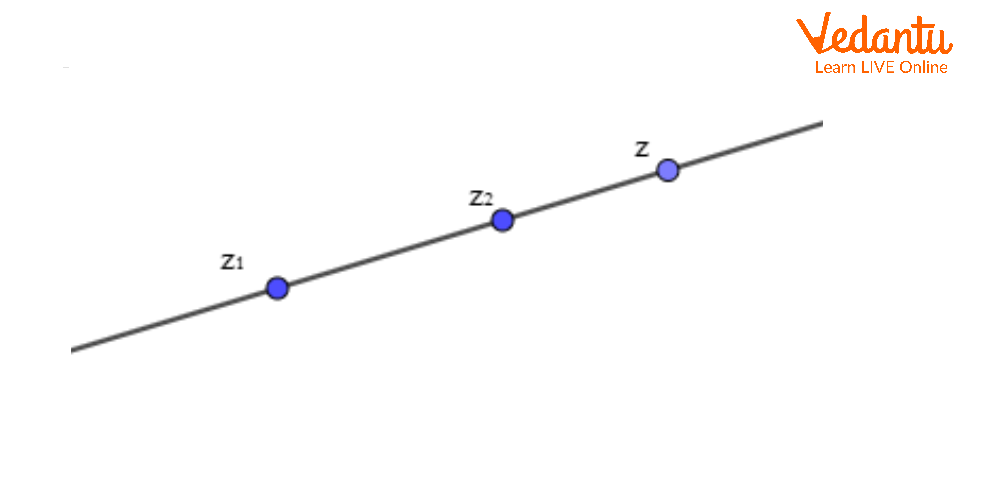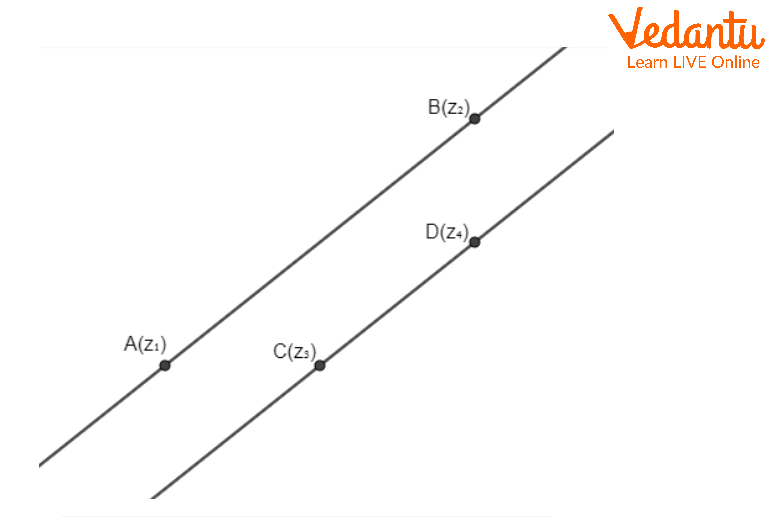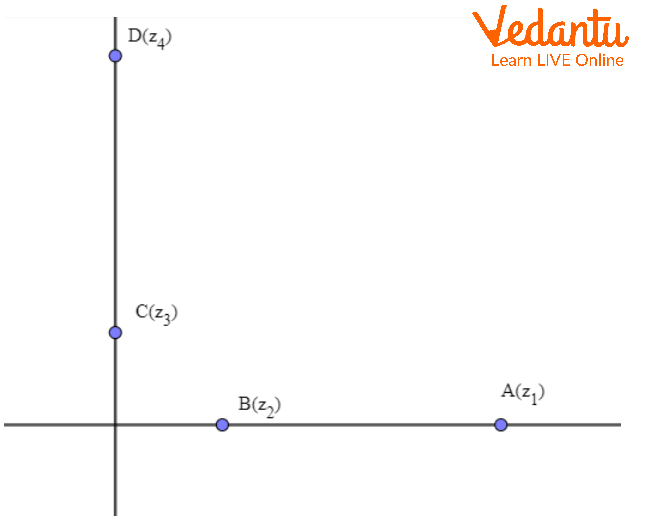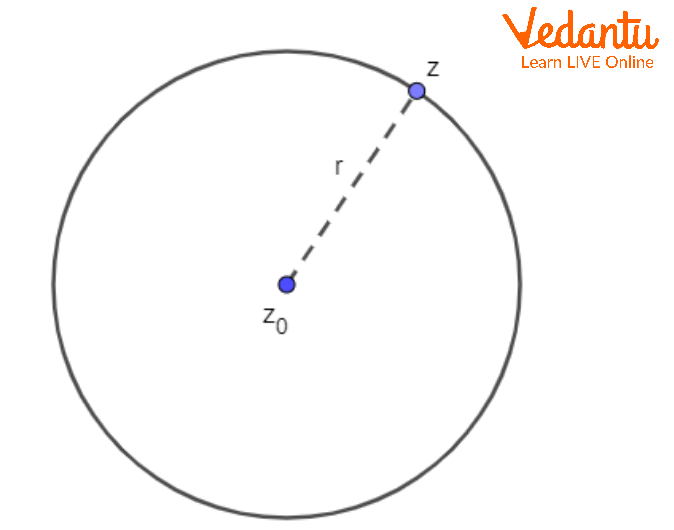Courses
Courses for Kids
Free study material
Free LIVE classes
More

# Straight Lines and Circles: JEE Important TopicLIVE
Join Vedantu’s FREE Mastercalss

## What are Straight Lines and Circles?

A straight line can be defined as a straight figure that is not curved having zero width. It can be represented by a linear equation, e.g., $ax+by+c=0$, where $a$ and $b$ are coefficients and $c$ is the constant. There are various forms of equations of a straight line that have been introduced in earlier classes, such as Point-slope form, Two-Point form, Slope-Intercept form.

A circle is defined as a two-dimensional shape that consists of all points in a plane that are equidistant from a fixed point, known as the centre of the circle.

The general equation of a circle having centre at (h,k) and radius equal to ‘r’ is given as ${{\left( x-h \right)}^{2}}+{{\left( y-k \right)}^{2}}={{r}^{2}}$. In complex plane, the equation of a straight line is given as $\left| \left. z-p \right| \right.=\left| \left. z-q \right| \right.$, where ‘p’  and ‘q’ are two distinct complex numbers.

The above line is bisecting the line segment joining the points p and q. It is the geometric way for the straight-line equation.

## Forms of Equations of a Straight Line

• Point-Slope Form- Let us consider a point $A\left( {{x}_{1}},\text{ }{{y}_{1}} \right)$ through which a line is passing and its slope be $m$. Then, the equation of the line is given as $y\text{ }-\text{ }{{y}_{1}}=\text{ }m\left( x\text{ }\text{-}{{x}_{1}} \right)$.

• Two-Point Form- Let us consider two fixed points as $A\left( {{x}_{1}},\text{ }{{y}_{1}} \right)$ & $B\text{ }\left( {{x}_{2}},\text{ }{{y}_{2}} \right)$. Then, the line joining these points can be represented by the equation $y-{{y}_{1}}=\frac{{{y}_{2}}-{{y}_{1}}}{{{x}_{2}}-{{x}_{1}}}\left( x-{{x}_{1}} \right).$

• Slope-Intercept Form- Let $c$ be the $y-$ intercept of a line, i.e., the line passes through a point (0, c). If the slope of the line is $m$, then, the equation of the line is given as $y\text{ }=\text{ }mx\text{ }+\text{ }c.$

• Intercept Form- Consider a line L with x-intercept a and y-intercept b; the line will intersect the X- axis at $(a,0)$ and the Y- axis at $(0,b)$. Then the equation of the line is given as:

$\dfrac{x}{a}+\dfrac{y}{b}=1$

• Normal Form: Consider a perpendicular from the origin to line L with length l that forms an angle with the positive X-axis. Then the equation of the line is given as:

$xcos\beta+ysin\beta=l$

## Equation of Straight Line in Complex Plane

A complex number is an expression of the form $z=x+iy$ where $x$ is the real part of $z$ and $y$ is the imaginary part.

The complex conjugate of $z=x+iy$ is defined as $\bar{z}=x-iy$

Now, we know that $z=x+iy$ and $\bar{z}=x-iy$.

Therefore, $x=\dfrac{z+\bar{z}}{2}$ and $y=\dfrac{z-\bar{z}}{2i}$

The general equation of a straight line is given as $ax+by+c=0$.

So, using the values of x and y, we can write the equation as

$a(\dfrac{z+\bar{z}}{2})+b(\dfrac{z-\bar{z}}{2i})+c=0$

As we know that $i^2 = -1$

$\Rightarrow az+a\bar{z}-bi(z-\bar{z})+2c=0$

$\Rightarrow z(a-bi)+\bar{z}(a+bi)+C=0$

Let $a'=a+bi$ and $\bar{a'}=a-bi$

Then, $\bar{a'}z+\bar{z}a’+C=0$  where $a’$ is a complex number and $C$ is a real number      …… (1)

The above equation is a general equation of a straight line in a complex plane.

The slope of the straight line $\bar{a'}z+\bar{z}a’+C=0$ is given as $-\dfrac{\text{cofficient of }\bar{z}}{\text{cofficient of }z}$, i.e., $\dfrac{-a’}{\bar{a’}}$.

## Parametric Equation of a Straight Line

Let ${{z}_{1}}$ and ${{z}_{2}}$ are two points in the complex plane. And z be any arbitrary point on the line joining the two points as shown below.A Line in Complex Plane

If we view the complex numbers as vectors, then $z-{{z}_{1}}$needs to be in the same direction as $z-{{z}_{2}}$. Then, $z-{{z}_{1}}$ can be obtained by multiplying $z-{{z}_{2}}$ with some scalar, say $\lambda$, i.e., $z-{{z}_{1}}=\lambda \left( z-{{z}_{2}} \right),$where $\lambda \epsilon \mathbb{R}$

$\Rightarrow z-\lambda z={{z}_{1}}-\lambda {{z}_{2}}$

$\Rightarrow z=(\frac{1}{1-\lambda }){{z}_{1}}-(\frac{\lambda }{1-\lambda }){{z}_{2}}$

$\Rightarrow z=t{{z}_{1}}+(1-t){{z}_{2}}$ where $t=\frac{1}{1-\lambda} \epsilon\mathbb{R}$   …… (2)

The above equation is a parametric form of a straight line in a complex plane.

## Condition for Two Lines to be Parallel

In Coordinate Geometry: If two straight lines with slope $m_1$ and $m_2$ are parallel to each other then Slope of both lines will be equal.

Therefore $m_1 = m_2$

In the Complex Plane: Let us consider two parallel lines as shown below.Two Parallel Lines in Complex Plane

Slope of line AB = $\dfrac{z_{1}-z_{2}}{\bar{z_{1}}-\bar{z_{2}}}$

Slope of line CD = $\dfrac{z_{3}-z_{4}}{\bar{z_{3}}-\bar{z_{4}}}$

Now, if the above two lines are parallel, then

Arg$\left(\dfrac{z_{1}-z_{2}}{{z_{3}}-{z_{4}}}\right)$ = $0$ and $\pi$

Since its argument is 0 and $\pi$ then this point will lie on a real axis. Therefore we can write using the condition $z= \bar{z}$,

$\left(\dfrac{z_{1}-z_{2}}{{z_{3}}-{z_{4}}}\right)=\left(\dfrac{\bar{z_{1}}-\bar{z_{2}}}{{\bar{z_{3}}}-{\bar{z_{4}}}}\right)$

$\dfrac{z_{1}-z_{2}}{\bar{z_{1}}-\bar{z_{2}}}$=$\dfrac{z_{3}-z_{4}}{\bar{z_{3}}-\bar{z_{4}}}$

Therefore, if ${{w}_{1}}$ and ${{w}_{2}}$ are slopes of two lines $AB$ and $CD$ respectively, then the condition for the lines to be parallel is ${{w}_{1}}={{w}_{2}}$.

## Condition for Two Lines to Be Perpendicular

In Coordinate Geometry: If two straight lines with slope $m_1$ and $m_2$ are perpendicular to each other then the relation between slope of both lines will be:

$m_1 \times m_2 = -1$

In the Complex Plane: Let us consider two perpendicular lines as shown below.Two Perpendicular Lines in Complex Plane

Slope of line AB = $\dfrac{z_{1}-z_{2}}{\bar{z_{1}}-\bar{z_{2}}}$

Slope of line CD = $\dfrac{z_{3}-z_{4}}{\bar{z_{3}}-\bar{z_{4}}}$

Now, if the above two lines are perpendicular, then

Arg$\left(\dfrac{z_{1}-z_{2}}{{z_{3}}-{z_{4}}}\right)$ = $\pm \dfrac{\pi}{2}$

Since its argument is  $\pm \dfrac{\pi}{2}$ then this point will lie on an imaginary axis. Therefore we can write,

$\left(\dfrac{z_{1}-z_{2}}{{z_{3}}-{z_{4}}}\right) + \left(\dfrac{\bar{z_{1}}-\bar{z_{2}}}{{\bar{z_{3}}}-{\bar{z_{4}}}}\right) = 0$

$\left(\dfrac{z_{1}-z_{2}}{{z_{3}}-{z_{4}}}\right) = - \left(\dfrac{\bar{z_{1}}-\bar{z_{2}}}{{\bar{z_{3}}}-{\bar{z_{4}}}}\right)$

$\dfrac{z_{1}-z_{2}}{\bar{z_{1}}-\bar{z_{2}}}$ = -   $\dfrac{z_{3}-z_{4}}{\bar{z_{3}}-\bar{z_{4}}}$

$\dfrac{z_{1}-z_{2}}{\bar{z_{1}}-\bar{z_{2}}}$ +   $\dfrac{z_{3}-z_{4}}{\bar{z_{3}}-\bar{z_{4}}} = 0$

Therefore, if ${{w}_{1}}$ and ${{w}_{2}}$ are slopes of two lines $AB$ and $CD$ respectively, then the condition for the lines to be perpendicular is ${{w}_{1}}+{{w}_{2}}=0$.

## Equation of Circle in Various Forms

• The Simplest equation of a circle is $x^2+y^2=r^2$ whose centre is (0,0) and radius is r.

• The equation $(x-a)^2+(y-b)^2=r^2$ represents a circle whose centre is (a,b) and radius is r.

• The general equation of a circle is $x^2+y^2+2gx+2fy+c=0$ whose centre is (-g,-f) and radius is $\sqrt{g^2+f^2+c}$.

• If point P(a,b) and Q(c,d) are endpoints of the diameter then the equation of circle is $(x-a)(x-c)+(y-b)(y-d) = 0$

• Parametric Equation of Circle: If the circle is given by $x^2+y^2=r^2$ then $x=r cos \theta$ and $y=r sin \theta$ are parametric equations.

## Circle- Equation in Complex Plane

A circle can be defined as the locus of points that are equidistant from a fixed point, i.e., centre; and the distance of the points from the centre is called the radius of the circle.

Let us consider a circle with centre at ${{z}_{0}}$ and $z$ be the locus of points on the circumference. Let $r$ be the radius of the circle as shown below in the diagram.A Circle in Complex Plane

The distance between the centre, ${{z}_{0}}$ and $z$ will always be equal to the radius $r$, i.e., $\left| z-{{z}_{0}} \right|=r.$

Squaring both the sides gives

$|z-z_{0}|^2=r^2$

$\Rightarrow (z-{{z}_{0}})(\overline{z-{{z}_{0}})}={{r}^{2}}$

$\Rightarrow z\bar{z}-z\overline{{{z}_{0}}}-\bar{z}{{z}_{0}}+|{{z}_{0}}{{|}^{2}}-{{r}^{2}}=0$

$\Rightarrow |z{{|}^{2}}+\bar{z}\alpha +z\bar{\alpha }+k=0$

where $\alpha=-z_{0}$ and $k=|z_{0}|^2-r^2$

The above equation is general equation of a circle in complex form, having centre as $-\alpha$ and radius will be $\sqrt{|z_{0}|^2-k}$.

## Summary

• The general equation of a straight line in a complex plane is given as $\bar{a'}z+\bar{z}a’+c=0$  where $a’$ is a complex number and $c$ is a real number.

• The parametric form of straight line in complex plane is given as $z=t{{z}_{1}}+(1-t){{z}_{2}}$ where $t=\frac{1}{1-\lambda} \epsilon\mathbb{R}$.

• The condition for two straight lines in complex plane to be parallel is given as  $w_{1}=w_{2}$ where ${{w}_{1}}$ and ${{w}_{2}}$ are slopes of lines $AB$ and $CD$ respectively.

• The condition for two straight lines in complex plane to be perpendicular is given as $w_{1}+w_{2}=0$, where ${{w}_{1}}$ and ${{w}_{2}}$ are slopes of lines $AB$ and $CD$ respectively.

• The general equation of a circle in complex form is given as $|z{{|}^{2}}+\bar{z}\alpha +z\bar{\alpha }+k=0$ where centre of the circle is $-\alpha$ and it’s radius is $\sqrt{|z_{0}|^2-k}$.

Last updated date: 21st Sep 2023
Total views: 116.7k
Views today: 2.16k

## FAQs on Straight Lines and Circles: JEE Important Topic

1. How can the distance between two points in a complex plane be determined?

The absolute value of any complex number $a+bi$ is given as

$\left| \left. a+bi \right| \right.=\sqrt{{{a}^{2}}+{{b}^{2}}}$

This also gives the distance between the origin and the point $\left( a,\text{ }b \right)$ in the complex plane. The distance between any two points in a complex plane is the absolute value of difference of the two complex numbers.

Let $\left( a,\text{ }b \right)$and $\left( p,q \right)$ are two arbitrary complex numbers in the complex plane. Then, the difference between these two numbers will be

$\left( p+qi \right)-\left( a+bi \right)=\left( p-a \right)+\left( q-b \right)i$

Then, the distance is determined as

$\left| \left. \left( p-a \right)+\left( q-b \right)i \right| \right.=\sqrt{{{\left( p-a \right)}^{2}}{{\left( q-b \right)}^{2}}}$

$\Rightarrow d=\sqrt{{{\left( p-a \right)}^{2}}{{\left( q-b \right)}^{2}}}$

The above expression gives the distance between any two points in the complex plane.

2. Why do we require imaginary numbers and Complex Planes?

The imaginary numbers, also known as complex numbers, have much importance in real-life. Their applications include concepts like electricity, some of quadratic equations (when the determinant is negative). In the cartesian planes, the imaginary numbers can’t be represented, therefore we need a different coordinate system or a different plane, that is the Complex plane. Imaginary numbers are widely used in Advanced Calculus and Physics.

Some of the examples are Study of Quantum Physics and modelling of periodic motions. In Mathematics, Euler’s equations, and various trigonometric equations require the use of imaginary numbers.